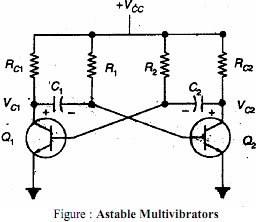Working of an astable multivibrator, Electrical Engineering

Assignment Help:

Q. With the help of a npn transistor circuit and wave forms explain the working of an astable multivibrator

In astable multivibrator both transistors are coupled to each other through capacitors as shown in the circuit diagram. Whichever transistor is off at any moment cannot remain off indefinitely; its base will become forward biased as that capacitor charges towards +5 volts.Once that happens, that transistor will turn on, thereby turning the other one off. If we pick a moment when Q1 has just turned off and Q2 is on, then the left end of C2 is at -5 volts. This negative voltage decreases as C2 charges through R2 towards +5 volts. However, the moment C2 charges enough to provide forward bias to the base of Q1, Q1 turns on and the 5 volt drop in Q1's collector voltage is coupled through C1 to the base of Q2. This turns Q2 off at once. As we saw in the previous experiment, the time that Q1 remains on and Q2 remains off is 0.693RC, which for the component values shown here is about 1 second.

Now Q2 is held off while C1 charges through R1, until Q2's base becomes forward biased. At that point the transistors switch states again and the whole thing starts over. There is no stable state where the circuit can come to rest, so this circuit is known as an astable multivibrator.

The time Q2 remains off is set by R1 and C1, just as the time Q1 remains off is set by R2 and C2. For our circuit, the components are of the same values on each side, so the timing will be the same on each half of the cycle. This is not required; the two halves of the circuit can have totally different time intervals. They actually operate independently of each other, even though they work together.

Since this particular circuit will spend about 1 second on each half cycle, the total cycle time, or period, is about 2 seconds. The operating frequency of the circuit is the reciprocal of the period, or 0.5 Hz.

Calculate the total power supplied to the divider circuit, There is often a...

There is often a need to produce more than one voltage from a single supply using a voltage divider. For example, the memory components of many personal computers require voltages

Explain the purpose of the i/o instructions in and out, Explain the purpose...

Explain the purpose of the I/O instructions IN and OUT. The IN instruction is used to move data from an I/O port into the accumulator. The OUT instruction is used to move d

Find the force between the wires, Q. A magnetic force exists between two ad...

Q. A magnetic force exists between two adjacent, parallel current-carryingwires. Let I1 and I2 be the currents carried by the wires, and r the separation between them.

Explain polymers and their applications, Explain Polymers and their applica...

Explain Polymers and their applications. Polymeric materials or plastics contain a large group of organo or organic metallic high molecules compound. The common properties of s

Find C and Rb

What is assembler, What is assembler? Assembler: An assembler or macr...

What is assembler? Assembler: An assembler or macro-assembler usually forms a part of the operating system. This translates an assembly language program into machine language

Find the antenna temperature, Q. If an antenna has an available noise power...

Q. If an antenna has an available noise power of 1.6 × 10 -15 W in a 1-MHz bandwidth, find the antenna temperature.

Transformer, 1 diagram basic layout of a simple transformer

1 diagram basic layout of a simple transformer

Clippers, Which clipper would you prefer between the series and the shunt c...

Which clipper would you prefer between the series and the shunt clipper? Why?

Butterworth filter, what is experimental setup for Butterworth filter?

what is experimental setup for Butterworth filter?